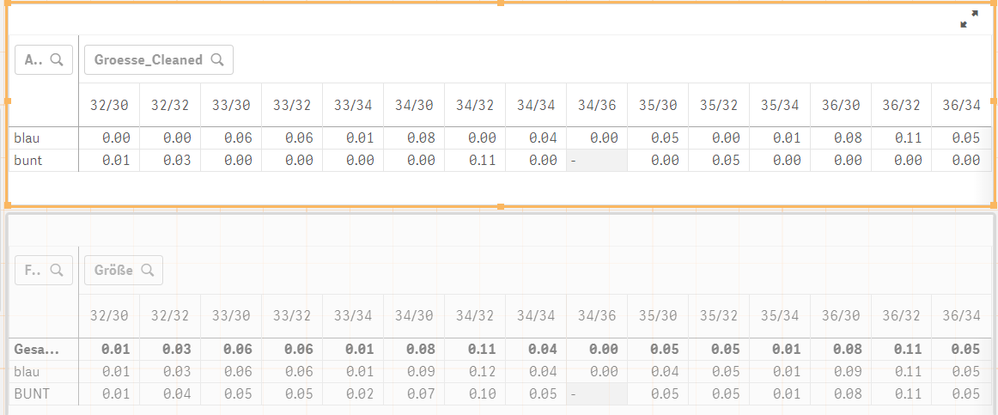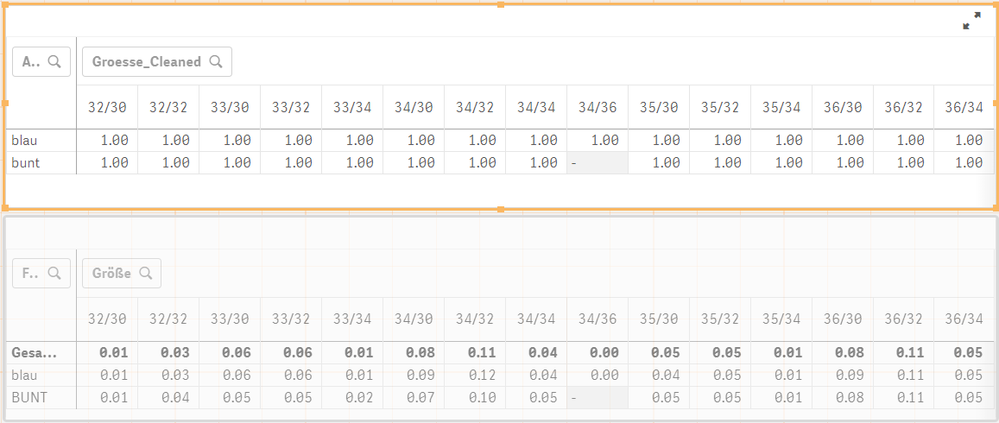# App Development

Announcements
Support Cases coming to Qlik Community Oct. 4! Start chats, open cases, explore resources: READ DETAILS
cancel
Showing results for
Did you mean:Contributor

## Calculate Total of Pivot Tabel Columns and Show it in Each Cell

Hello,

I am working with pivot table and want to set values NULL in dependents of the total of the columns. The dimensions of my table are the color and size of products. In my approach I try to

1. calculate the total by myself...
2. ... and make it accessible in each cell of the column.
1. works fine, but I fail at 2.

The formula in the pivot table is

sum(Sales)/RangeSum(First(Sum(Sales), 1, NoOfColumns()))

and I calculate the total as

sum(aggr(sum(Sales), Sizes))/sum(total Sales)

However I end up with the followingThe marked is a pivot table to demonstrate my problem and contains my custom calculated total values. The bottom table I my actual pivot table with totals in bold text. As one can see I calculate the totals correctly but they are somehow randomly distributed in terms of row location.

Depending on the column I want the same value for each cell. For example, the correct values for 32/32 in the orange table are 0.03, 0.03 and not 0.00, 0.03.

I there a method to provid the totals to each cell? Is there a smater way to set columns to Null if the total is <=0.01?

Edit:

I updated the formula for the pivot table, because I forgot that I use RangeSum(First(Sum(Sales),1,NoOfColumns())) in the dominator and not just sum(total Sales).

8 RepliesMVP

May be this

``Sum(TOTAL <Size> Sales)/Sum(TOTAL Sales)``Contributor
Author

@sunny_talwarunfortunately your formula is a total "too much" and the set analysis does not work as desired:MVP

Size is one of the dimension within the chart?Contributor
Author

@sunny_talwar The dimensions are text(Size) for the columns and Color for the rows, but using <text(Size)> in the set analysis throws an error.MVP

May be you need square brackets

``<[text(Size)]>``Contributor
Author

@sunny_talwar  Unfortunatelys, the formula

sum(total <[text(SIZE)]> Sales)/sum(total Sales)

throws an error as well.MVP

What error message do you see?Partner

Hendrik,

it's not helping monday ,but it doesn't matter 🙂

to get the same calculation is each cell you easily take the formular:

sum(total <GROESSE_CLEANED> ABSATZ_STK)/sum(total ABSATZ_STK)

Hope it helps,

Cheers,

ChrisTags
Community Browser